# Graphical solving of equations

Approximate solution of equations. Graphical solving of
equations in one unknown. Graphical solving of systems
of simultaneous equations in two unknowns.

Graphical representation of functions permits to solve approximately any equation in one unknown and a system of two simultaneous equations in two unknowns. To solve a system of two simultaneous equations in two unknowns x and y graphically, we consider each of the equations as a functional dependence between variables x and y and build graphs for these two functions. Coordinates of intersection points of these graphs give us the found values for x and y ( i.e. a solution of this system of simultaneous equations ).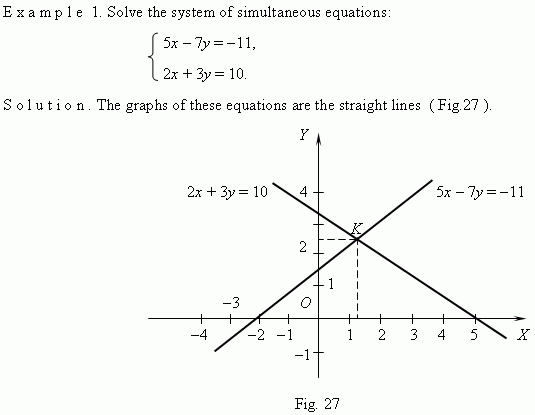According to these graphs the approximate coordinates of the intersection point K are: x = 1.25, y = 2.5.  The exact solution of these simultaneous equations is: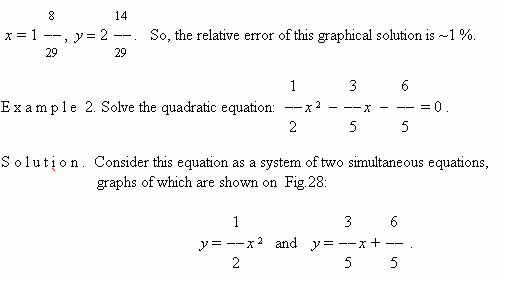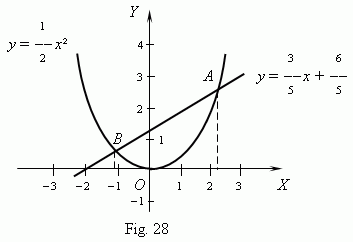After building the graphs we find abscissas of the intersection points A and B :
x 1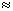2.25 , x 2–1.1 . Exact values of this equation roots are: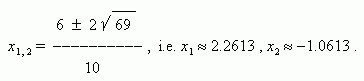The relative error of the graphical solution in this example is ~3.5 %.

To solve an equation in one unknown graphically, it is necessary to transfer all its terms to the same part, i.e. to reduce it to a shape:

f ( x ) = 0 ,

and to build a graph of the function  y = f ( x ). Abscissas of the graph intersection points with an x -axis will be roots of this equation (zeros of this function).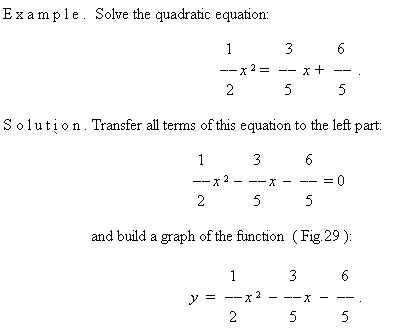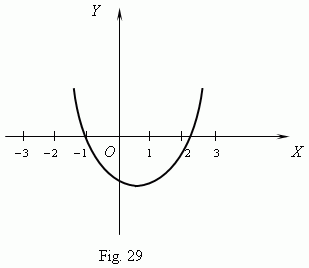By this graph we find zeros of the function: x 12.25, x 2–1.1.